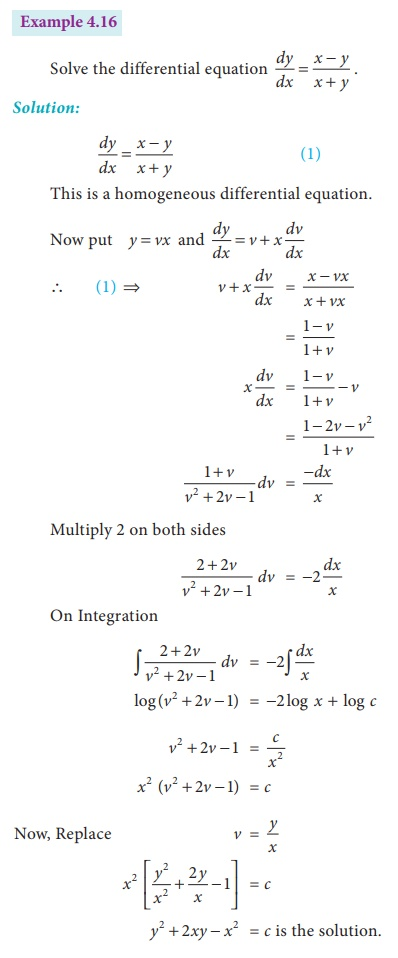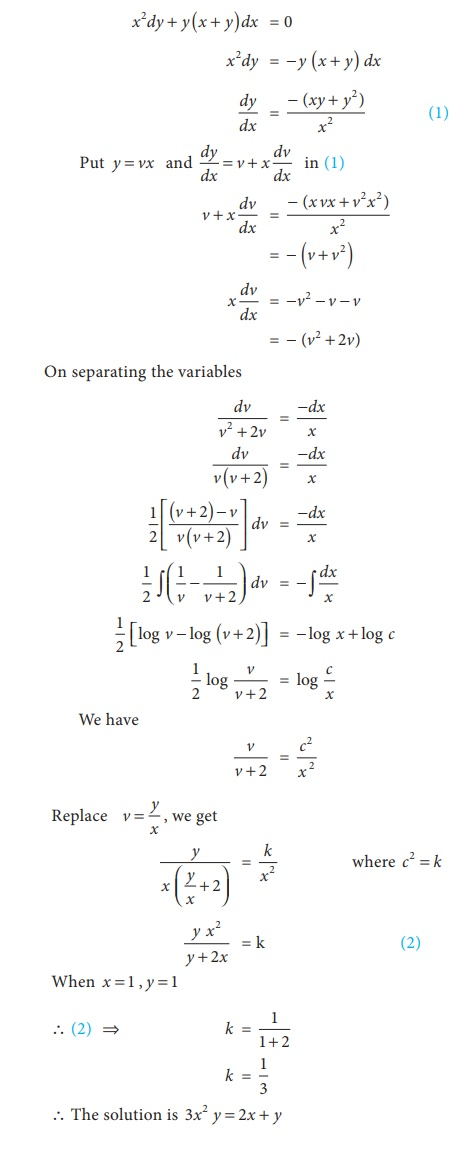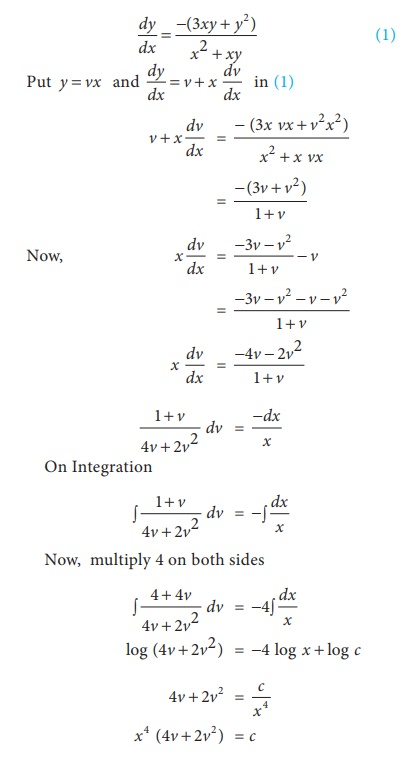Home | | Business Maths 12th Std | Homogeneous Differential Equations: Solved Example Problems

# Homogeneous Differential Equations: Solved Example Problems

Maths: Differential Equations: Homogeneous Differential Equations: Solved Example Problems with Answers, Solution and Explanation

Example 4.15

Solve the differential equation y2 dx + ( xy + x2 )dy = 0

SolutionExample 4.17

Find the particular solution of the differential equation x 2 dy + y ( x + y) dx = 0 given that x = 1, y = 1

Solution:Example 4.18

If the marginal cost of producing x shoes is given by (3xy + y2 ) dx + (x 2 + xydy = 0 and the total cost of producing a pair of shoes is given by ₹12. Then find the total cost function.

Solution:

Given marginal cost function is (x2 + xy) dy + (3xy + y2)dx=0Example 4.19

The marginal revenue ‘y’ of output ‘q’ is given by the equation. Find the total Revenue function when output is 1 unit and Revenue is ₹5.

Solution:Tags : with Answer, Solution, Formula , 12th Business Maths and Statistics : Chapter 4 : Differential Equations
Study Material, Lecturing Notes, Assignment, Reference, Wiki description explanation, brief detail
12th Business Maths and Statistics : Chapter 4 : Differential Equations : Homogeneous Differential Equations: Solved Example Problems | with Answer, Solution, Formula